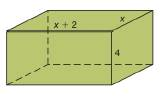Chapter 9.1, Problem 25E### Elementary Geometry for College St...

6th Edition
Daniel C. Alexander + 1 other
ISBN: 9781285195698

#### Solutions

Chapter
Section### Elementary Geometry for College St...

6th Edition
Daniel C. Alexander + 1 other
ISBN: 9781285195698
Textbook Problem
1 views

# For the box shown, the total area is 94 cm2. Determine the value of x .To determine

To find:

The value of ‘x’ for the box with its total area 94 cm2.

Explanation

The total area of any solid is the area of all the number of lateral sides that it have, including the area of the top and the bottom of solid.

Thus, the total area (T) of any solid prism with its lateral area (L) and base area (B) is given by the formula.

T=L+2B

Here, we have 2B since, any solid prison will have two bases (One upper part and another the lower part).

Calculation:

Given,

Total area of the box =94 cm2

Also from the given figure, the length ‘l’, width ‘w’, and the height ‘h’ of the box is given by,

l=x+2

w=x

h=4 cm also

T=94 cm

The total area of the cuboidal box is given by the formula

T =L+2B

=2lh+2wh+2lw

=2lh+wh+2lw

=2lh+wh+lw

T =2(lw+wh+hl)

94=2x+2x+x4+4x+2

94=2x2+2x+4x+4x+8

Combine, the like terms together

94=2x2+10x+8

Divide; by 2 on the both sides,

942=2x2</

### Still sussing out bartleby?

Check out a sample textbook solution.

See a sample solution

#### The Solution to Your Study Problems

Bartleby provides explanations to thousands of textbook problems written by our experts, many with advanced degrees!

Get Started

#### Solve the equations in Exercises 126. 1x9x3=0

Finite Mathematics and Applied Calculus (MindTap Course List)

#### Convert from radians to degrees. 9. 512

Single Variable Calculus: Early Transcendentals, Volume I

#### Use the following matrices for Problems 1-28. 4. Write the negative of D.

Mathematical Applications for the Management, Life, and Social Sciences

#### Evaluate the integral, if it exists. 03x24dx

Single Variable Calculus: Early Transcendentals

#### 0 1 2 ∞

Study Guide for Stewart's Single Variable Calculus: Early Transcendentals, 8th